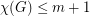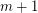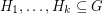# Alon-Saks-Seymour Conjecture (Solved)

 Importance: High ✭✭✭
 Author(s): Alon, Noga Saks, Michael Seymour, Paul D.
 Subject: Graph Theory » Coloring » » Vertex coloring
 Keywords: coloring complete bipartite graph eigenvalues interlacing
 Posted by: mdevos on: May 28th, 2007
 Solved by: Huang and Sudakov
Conjecture   Ifis a simple graph which can be written as an union ofedge-disjoint complete bipartite graphs, then.

This conjecture may be viewed as a bipartite analogue of the Erdos-Faber-Lovasz Conjecture.

One very special case of this conjecture is the statement that a complete graph onvertices cannot be written as a disjoint union of fewer thancomplete bipartite graphs (although it does have numerous such decompositions). This was proved by Graham and Pollak [GP] who used spectral techniques to establish the following stronger fact.

Theorem   Letbe a simple loopless graph, and assume that the adjacency matrix ofhaspositive andnegative eigenvalues. Ifis a list of edge disjoint subgraphs with unionand eachis a complete bipartite graph, then.

Alternative proofs of the Graham-Pollak Theorem have been found by [P] and [T], and Alon, Brualdi, and Schader [ABS] resolved a conjecture of de Caen by proving that wheneveris edge-colored so that each color class induces a complete bipartite graph, there is a spanning tree with one edge of each color. However, all of these results depend heavily on spectral tools. A proof of the general conjecture would appear to either require a vast generalization of these techniques, or a new combinatorial proof of the Graham-Pollak Theorem.

## Bibliography

[ABS] N. Alon, R. A. Brualdi and B. L. Shader, Multicolored forests in bipartite decompositions of graphs, J. Combinatorial Theory Ser. B 53 (1991), 143-148.

[GP] R. L. Graham and H. O. Pollak, On the addressing problem for loop switching, Bell Syst. Tech. J. 50 (1971), 2495-2519.

[P] G. W. Peck, A new proof of a theorem of Graham and Pol lak, Discrete Math. 49 (1984), 327-328.

[T] H. Tverberg, On the decomposition of Kn into complete bipartite graphs, J. Graph Theory 6 (1982), 493-494.

* indicates original appearance(s) of problem.

### Solved!!

Hao Huang and Benny Sudakov have found a fascinating counterexample to this conjecture. Their paper can be found on the arxiv here